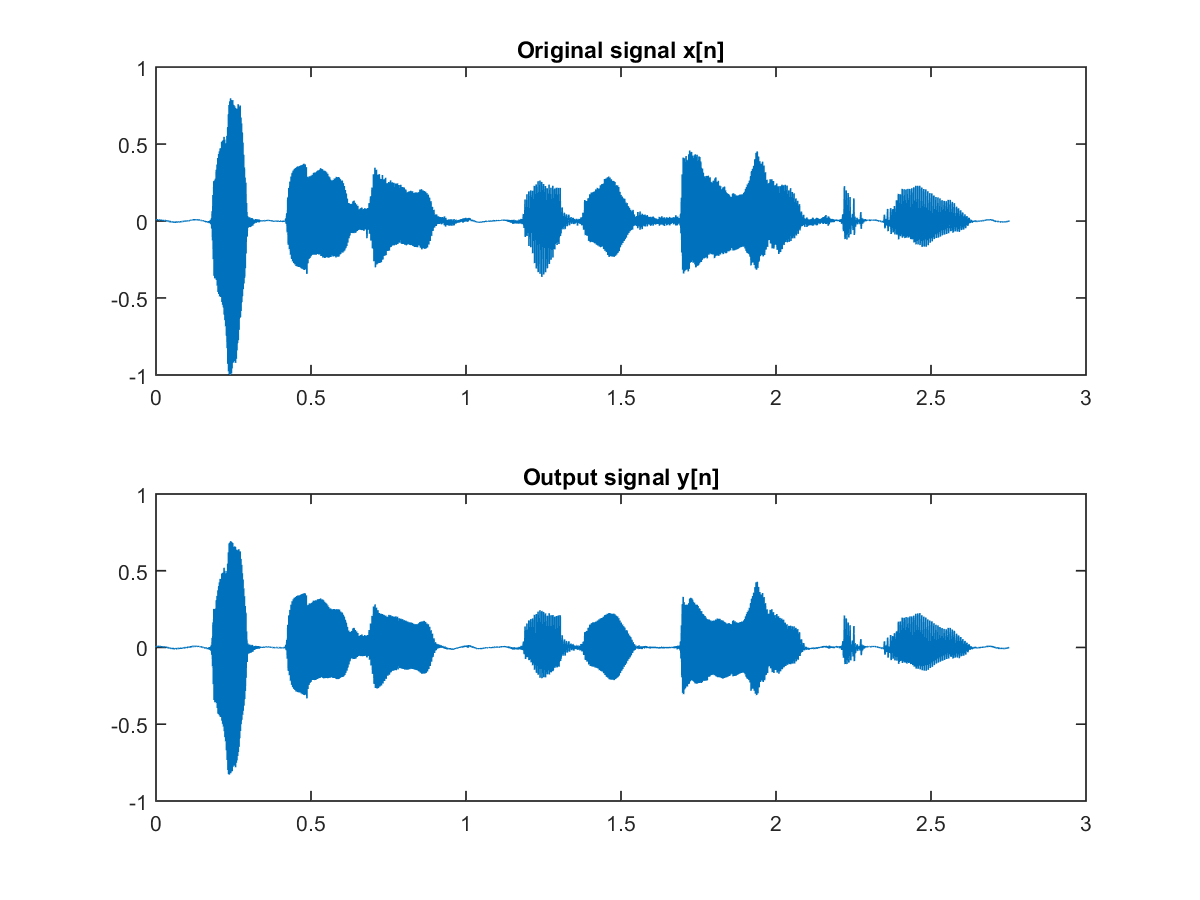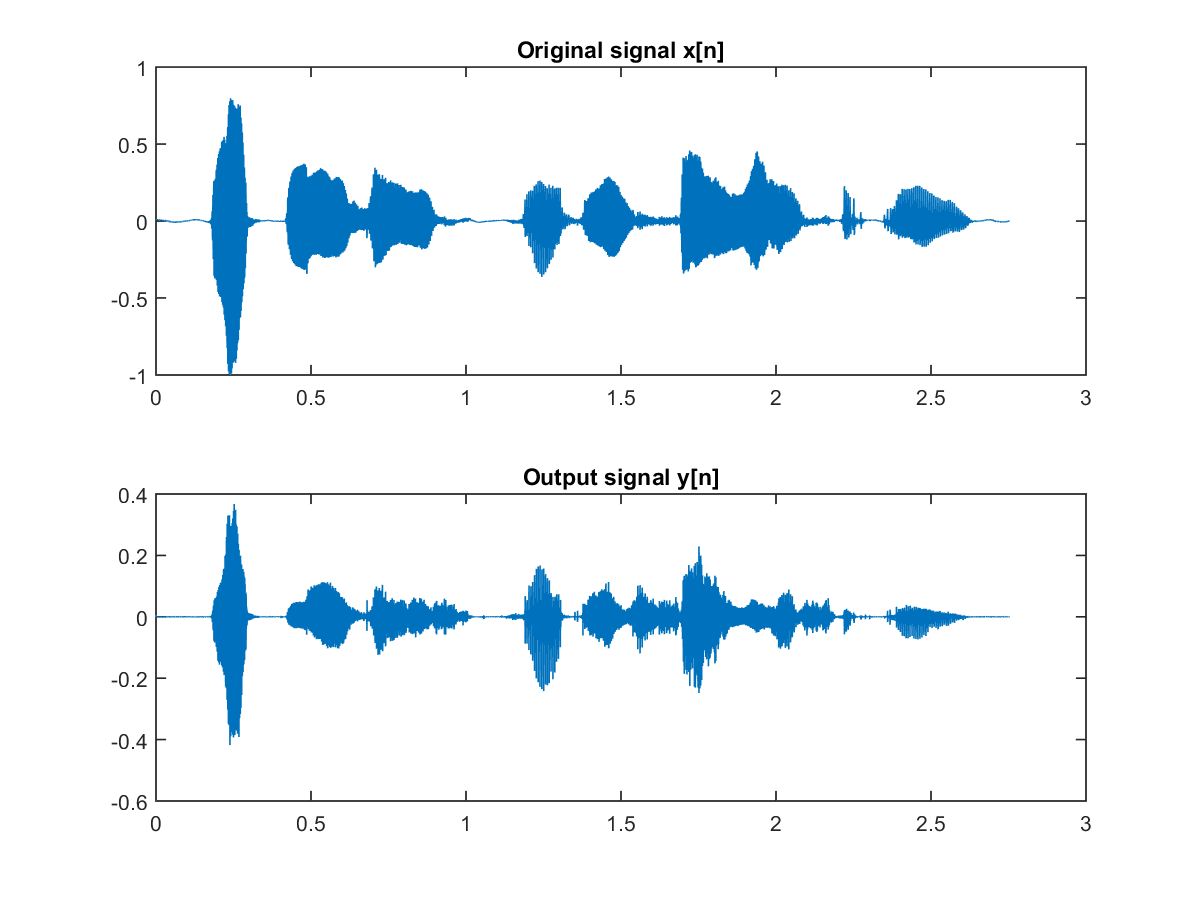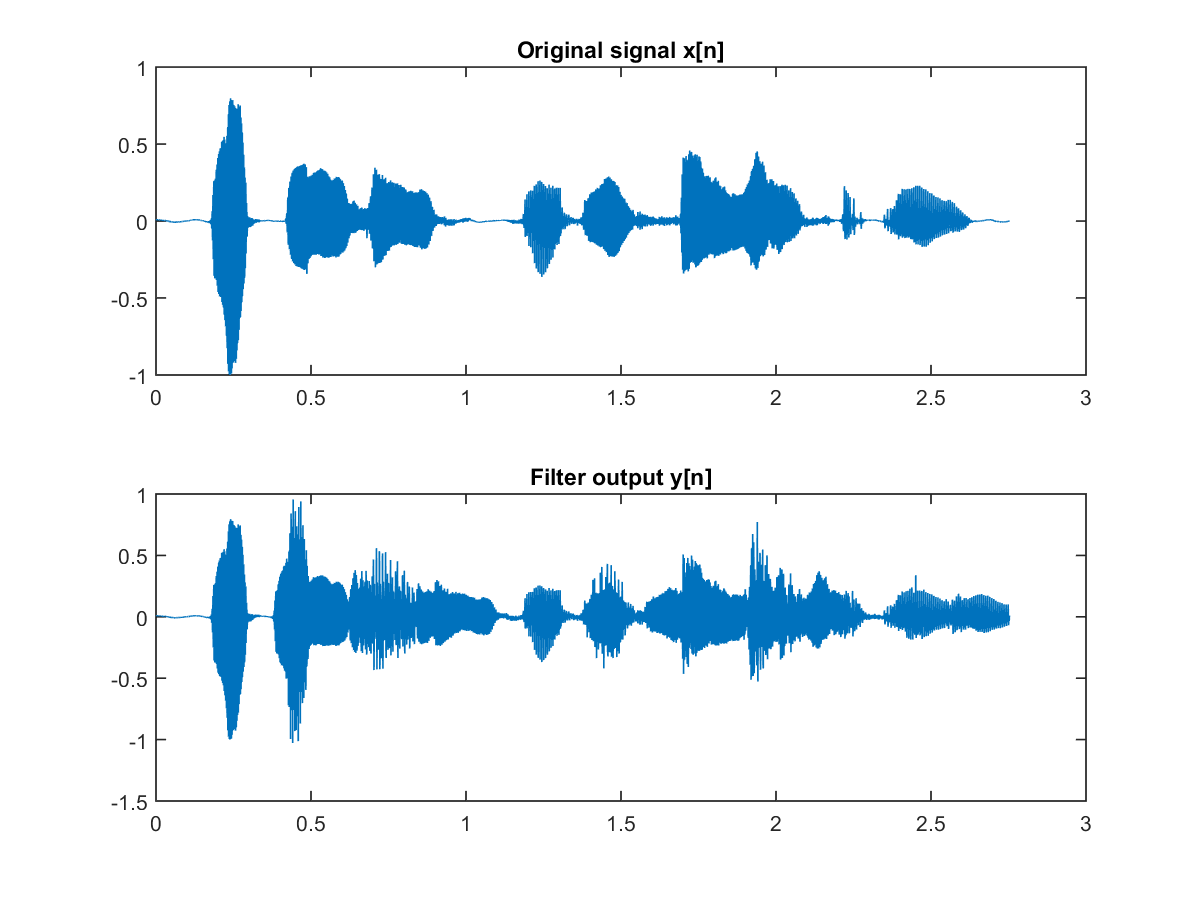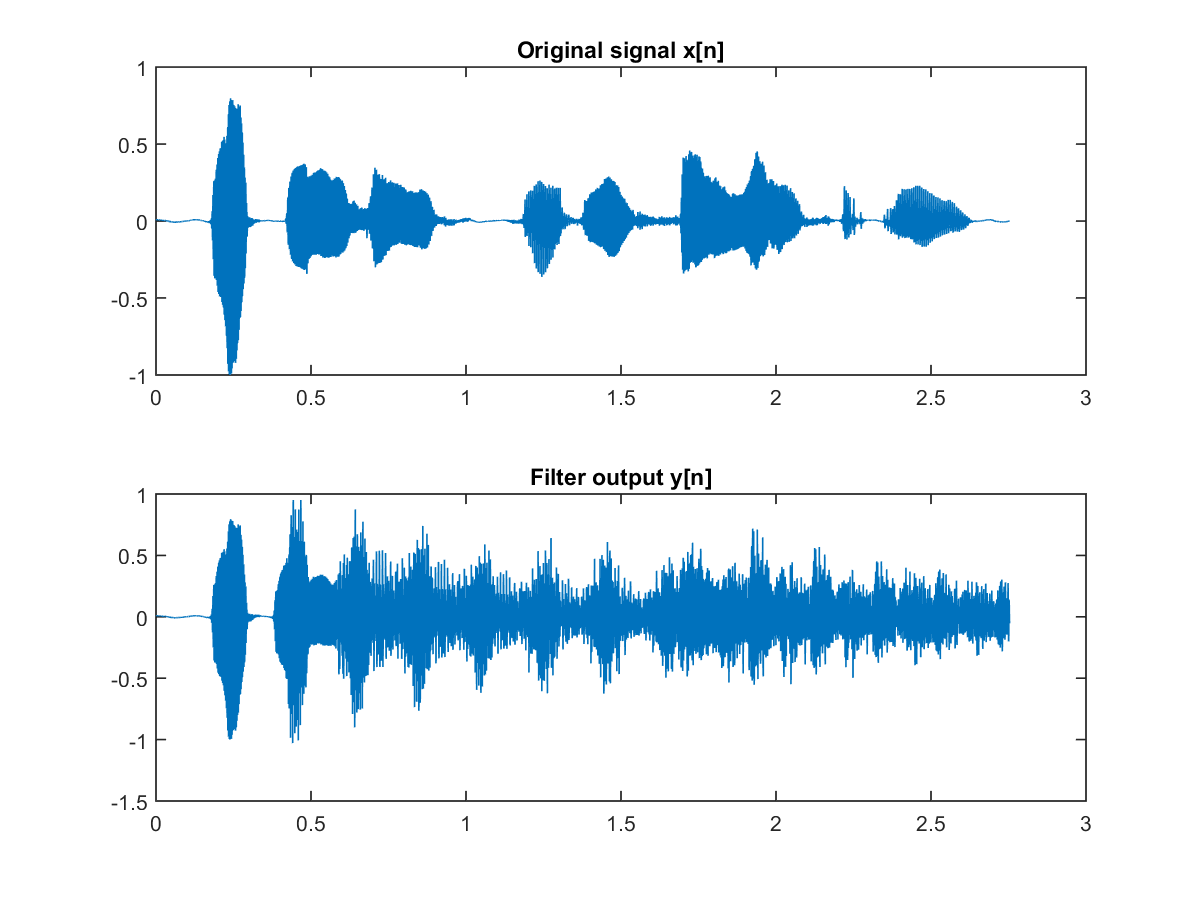## 11-1 Filter Applications (嚙踝蕭嚙踝蕭嚙踝蕭嚙踝蕭嚙踝蕭嚙踝蕭嚙踝蕭嚙?

In this section, we shall introduce the concept of digital filters and their applications. Most students who have taken DSP (digital signal processing) are more or less intimidated by the complexity of the underlying mathematics of digital filters. However, the focus of this section is on the applications of digital filters using MATLAB and the treatment should be pretty fundamental for most engineering-majored students.

To put it simply, a digital filter can be represented by two parameter vectors a and b, where the lenghts of a and b are p and q, respectively, and the first element of a is always 1, as follows:

a = [1, a2, ... ap]
b = [b1, b2, ... bq]
If we apply a digital filter with parameters a and b to a stream of discrete-time signal x[n], the output y[n] should satisfy the following equation:
y[n] + a2y[n-1] + a3y[n-2] + ... + apx[n-p+1] = b1x[n] + b2x[n-1] + ... + bqx[n-q+1]
Or equivalently, we can express y[n] explicitly:
y[n] = b1x[n] + b2x[n-1] + ... + bqx[n-q+1] - a2y[n-1] - a3y[n-2] - ... - apx[n-p+1]
The preceding equation seems a little bit complicated. We shall give some more specific examples to make it more easily understood.

First of all, if we have a filter with the parameters:

a = 
b = [1/5, 1/5, 1/5, 1/5, 1/5]
Then the output of the filter is
y[n] = (x[n] + x[n-1] + x[n-2] + x[n-3] + x[n-4])/5
This is a simple digital filter that sets y[n] as the average of the preceding five points of the input signals. In fact, this is the so-called low-pass filter since after the averaging operator, the high-frequency component is averaged out while the low-frequency component is more or less retained. The effect of a low-pass filter is like putting a paper cup on the mouth while speaking, generating a murmuring-like indistinct sound.

Hint
Why putting a paper cup on the mouth makes it a low-pass filter? Can you think of an intuitive reason?

The next example uses a low-pass filter on a speech input.

Example 1: lpf01.mwaveFile='whatMovies.wav'; au=myAudioRead(waveFile); % Filter parameters a = ; b = [1, 1, 1, 1, 1]/5; y = filter(b, a, au.signal); % Plot the result time = (1:length(au.signal))/au.fs; subplot(2,1,1); plot(time, au.signal); title('Original signal x[n]'); subplot(2,1,2); plot(time, y); title('Output signal y[n]'); au.signal=y; myAudioWrite(au, 'lpf01.wav'); % Save the output signalIn the above example, "y = filter(b, a, x)" is the MATLAB command to generate the output signal y from the original signal x and the filter's parameters a and b. From the waveform plots, we can also observe that the high-frequency components (especially the unvoiced sounds, such as "s" in "movies" and "seen", and "c" in "recently") have a smaller amplitude after filtering. This is a typical effect of low-pass filters. We can also hear the original and the output signals:
Let us take a look at another filter with the parameters:
a = 
b = [1, -1]
The output of the fitler is
y[n] = x[n] - x[n-1]
In other words, the output of the filter y[n] is equal to the difference of the preceding two points of x[n]. As the result, low-frequency components (with slow variations) will have low values while high-frequency components (with fast variations) will have high values. So this is a typical high-pass filter which amplifies high-frequency and suppresses low-frequency components. See the following example.

Example 2: hpf01.mwaveFile='whatMovies.wav'; au=myAudioRead(waveFile); % Filter parameters a = ; b = [1, -1]; y = filter(b, a, au.signal); % Plot the result time = (1:length(au.signal))/au.fs; subplot(2,1,1); plot(time, au.signal); title('Original signal x[n]'); subplot(2,1,2); plot(time, y); title('Output signal y[n]'); au.signal=y; myAudioWrite(au, 'hpf01.wav'); % Save the output signalFrom the waveform plots, we can observe that the amplitudes of the unvoiced sounds (high-frequency components) are relatively larger that of the voiced sounds (low-frequency components). We can also hear the sound clips directly:
After the high-pass filter, the output signal is like the output from a small radio set with noise-like creaky sounds.

Besides low-pass and high-pass, digital filters can also produce some other familiar sound effects. For instance, if a filter has the following parameters:

a = 
b = [1, 0, 0, 0, ..., 0, 0.8] (That is, 3199 zeros between 1 and 0.8.)
Then the output of the filter is:
y[n] = x[n] + 0.8*x[n-3200]
In other words, this filter can produce "one-fold echo" for the given input signal. If the sample frequency fs is 16kHz, then the time difference between the input and the echo is 3200/fs = 3200/16000 = 0.2 second. Please see the following example.

Example 3: echo01.mwaveFile='whatMovies.wav'; au=myAudioRead(waveFile); % Filter parameters delay=0.2; gain=0.8; a = ; b = [1, zeros(1, round(delay*au.fs)-1), gain]; y = filter(b, a, au.signal); % Plot the result time = (1:length(au.signal))/au.fs; subplot(2,1,1); plot(time, au.signal); title('Original signal x[n]'); subplot(2,1,2); plot(time, y); title('Filter output y[n]'); au.signal=y; myAudioWrite(au, 'echo01.wav'); % Save the output signal[Warning: Data clipped when writing file.] [> In <a href="matlab:matlab.internal.language.introspective.errorDocCallback('audiowrite>clipInputData', 'E:\MATLAB\R2015a\toolbox\matlab\audiovideo\audiowrite.m', 396)" style="font-weight:bold">audiowrite>clipInputData</a> (<a href="matlab: opentoline('E:\MATLAB\R2015a\toolbox\matlab\audiovideo\audiowrite.m',396,0)">line 396</a>) In <a href="matlab:matlab.internal.language.introspective.errorDocCallback('audiowrite', 'E:\MATLAB\R2015a\toolbox\matlab\audiovideo\audiowrite.m', 176)" style="font-weight:bold">audiowrite</a> (<a href="matlab: opentoline('E:\MATLAB\R2015a\toolbox\matlab\audiovideo\audiowrite.m',176,0)">line 176</a>) In <a href="matlab:matlab.internal.language.introspective.errorDocCallback('myAudioWrite', 'd:\users\jang\matlab\toolbox\sap\myAudioWrite.m', 29)" style="font-weight:bold">myAudioWrite</a> (<a href="matlab: opentoline('d:\users\jang\matlab\toolbox\sap\myAudioWrite.m',29,0)">line 29</a>) In <a href="matlab:matlab.internal.language.introspective.errorDocCallback('echo01', 'D:\users\jang\books\audioSignalProcessing\example\echo01.m', 16)" style="font-weight:bold">echo01</a> (<a href="matlab: opentoline('D:\users\jang\books\audioSignalProcessing\example\echo01.m',16,0)">line 16</a>) In <a href="matlab:matlab.internal.language.introspective.errorDocCallback('goWriteOutputFile>dummyFunction', 'D:\users\jang\books\goWriteOutputFile.m', 85)" style="font-weight:bold">goWriteOutputFile>dummyFunction</a> (<a href="matlab: opentoline('D:\users\jang\books\goWriteOutputFile.m',85,0)">line 85</a>) In <a href="matlab:matlab.internal.language.introspective.errorDocCallback('goWriteOutputFile', 'D:\users\jang\books\goWriteOutputFile.m', 55)" style="font-weight:bold">goWriteOutputFile</a> (<a href="matlab: opentoline('D:\users\jang\books\goWriteOutputFile.m',55,0)">line 55</a>)]In the above example, gain = 0.8 is the decay ratio of the echo, and delay = 0.2 is the delay time of the echo. We can hear the sound clips:
The above filter can only produce one-fold echo. If we want to have a more realistic multiple-fold echo, we can use the following parameters:
a = [1, 0, 0, 0, ..., 0, -0.8] (That is, 3199 zeros between 1 and -0.8.)
b = 
The output of the filter is
y[n] = x[n] + 0.8*y[n-3200]
The filter can produce a more realistic multiple-fold echo. If the sample frequency fs = 16kHz, then the time delay between echos is 3200/fs = 3200/16000 = 0.2 秒. See the following example.

Example 4: echo02.mwaveFile='whatMovies.wav'; au=myAudioRead(waveFile); % Filter parameters delay=0.2; gain=0.8; a = [1 zeros(1, round(delay*au.fs)), -gain]; b = ; y = filter(b, a, au.signal); % Plot the result time = (1:length(au.signal))/au.fs; subplot(2,1,1); plot(time, au.signal); title('Original signal x[n]'); subplot(2,1,2); plot(time, y); title('Filter output y[n]'); au.signal=y; myAudioWrite(au, 'echo02.wav'); % Save the output signal[Warning: Data clipped when writing file.] [> In <a href="matlab:matlab.internal.language.introspective.errorDocCallback('audiowrite>clipInputData', 'E:\MATLAB\R2015a\toolbox\matlab\audiovideo\audiowrite.m', 396)" style="font-weight:bold">audiowrite>clipInputData</a> (<a href="matlab: opentoline('E:\MATLAB\R2015a\toolbox\matlab\audiovideo\audiowrite.m',396,0)">line 396</a>) In <a href="matlab:matlab.internal.language.introspective.errorDocCallback('audiowrite', 'E:\MATLAB\R2015a\toolbox\matlab\audiovideo\audiowrite.m', 176)" style="font-weight:bold">audiowrite</a> (<a href="matlab: opentoline('E:\MATLAB\R2015a\toolbox\matlab\audiovideo\audiowrite.m',176,0)">line 176</a>) In <a href="matlab:matlab.internal.language.introspective.errorDocCallback('myAudioWrite', 'd:\users\jang\matlab\toolbox\sap\myAudioWrite.m', 29)" style="font-weight:bold">myAudioWrite</a> (<a href="matlab: opentoline('d:\users\jang\matlab\toolbox\sap\myAudioWrite.m',29,0)">line 29</a>) In <a href="matlab:matlab.internal.language.introspective.errorDocCallback('echo02', 'D:\users\jang\books\audioSignalProcessing\example\echo02.m', 16)" style="font-weight:bold">echo02</a> (<a href="matlab: opentoline('D:\users\jang\books\audioSignalProcessing\example\echo02.m',16,0)">line 16</a>) In <a href="matlab:matlab.internal.language.introspective.errorDocCallback('goWriteOutputFile>dummyFunction', 'D:\users\jang\books\goWriteOutputFile.m', 85)" style="font-weight:bold">goWriteOutputFile>dummyFunction</a> (<a href="matlab: opentoline('D:\users\jang\books\goWriteOutputFile.m',85,0)">line 85</a>) In <a href="matlab:matlab.internal.language.introspective.errorDocCallback('goWriteOutputFile', 'D:\users\jang\books\goWriteOutputFile.m', 55)" style="font-weight:bold">goWriteOutputFile</a> (<a href="matlab: opentoline('D:\users\jang\books\goWriteOutputFile.m',55,0)">line 55</a>)]We can hear the sound clips:

Audio Signal Processing and Recognition (音訊處理與辨識)